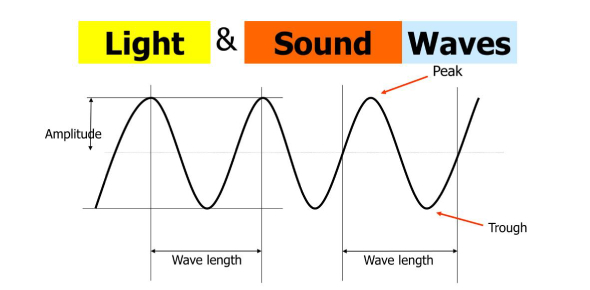# Waves, Light And Sound! Physics Trivia Quiz

22 Questions | Total Attempts: 132SettingsWhat do you know about waves, light, and sound? A sound wave is regarded as a longitudinal wave, while light waves are transverse waves. A Sound wave can be reflected and refracted just as in soft waves and can give rise to interface phenomena. However, no polarization can be accomplished with sound waves, unlike light waves. If you want to learn more about waves, light, and sound, this is the quiz for you.

• 1.
What property of waves is shown in the animation?
• 2.
In this animation, what type of wave is shown?
• A.

Transverse

• B.

Refracted

• C.

Longitudinal

• D.

Light

• 3.
The type of wave shown is a __________ wave.
• 4.
Which of the following waves transfer energy?
• A.

Sound

• B.

Light

• C.

Sea waves

• D.

Microwaves

• E.

All of the above

• 5.
All waves except __________ waves must travel through a medium.
• A.

Sound

• B.

Light

• C.

Water

• D.

Compressional

• 6.
Points A and F on the wave are ________
• 7.
Points D and I on the wave are __________________.
• 8.
The distance between A and F is the _____________.
• A.

Frequency

• B.

Period

• C.

Amplitude

• D.

Wavelength

• 9.
The distance from the dotted line to Point A, or the distance from the dotted line to Point D is known as the ______________.
• A.

Frequency

• B.

Period

• C.

Amplitude

• D.

Wavelength

• 10.
this image shows an example of:
• A.

Reflection

• B.

Refraction

• C.

Penumbra

• D.

A broken straw

• 11.
The wave speed equation is s = frequency x wavelength. If the speed of the wave is 400 m/s  and the wavelength is 2m, what is its frequency?
• A.

200 Hz

• B.

800 Hz

• C.

200 m/s

• D.

400 Hz

• 12.
What do waves P and Q have in common?
• A.

Same Period

• B.

Same Frequency

• C.

Same Amplitude

• D.

All of the above

• 13.
Which wave has the lowest amplitude?
• A.

P

• B.

S

• C.

Q

• D.

R

• 14.
Which wave has changing frequency?
• A.

S

• B.

Q

• C.

R

• D.

P

• 15.
A wave has a period of 10s and a wavelength of 2m. What is its speed? write the answer with the proper unit (Do not use space in your answer)
• 16.
Name the region labeled with I:
• A.

Compression

• B.

Rarefaction

• C.

Wavelength

• D.

Frequency

• 17.
Name the region labeled with H:
• A.

Compression

• B.

Rarefaction

• C.

Wavelength

• D.

Frequency

• 18.
Name the region labeled with G:
• A.

Compression

• B.

Rarefaction

• C.

Wavelength

• D.

Frequency

• 19.
What type of wave vibrates parallel to the direction of travel?
• 20.
In what type of wave is the vibration perpendicular to the direction of travel of the wave?
• 21.
What type of wave contains compression and rarefaction?
• 22.
What is the phenomena when a wave, like light, bounces off an object.
Related TopicsBack to top